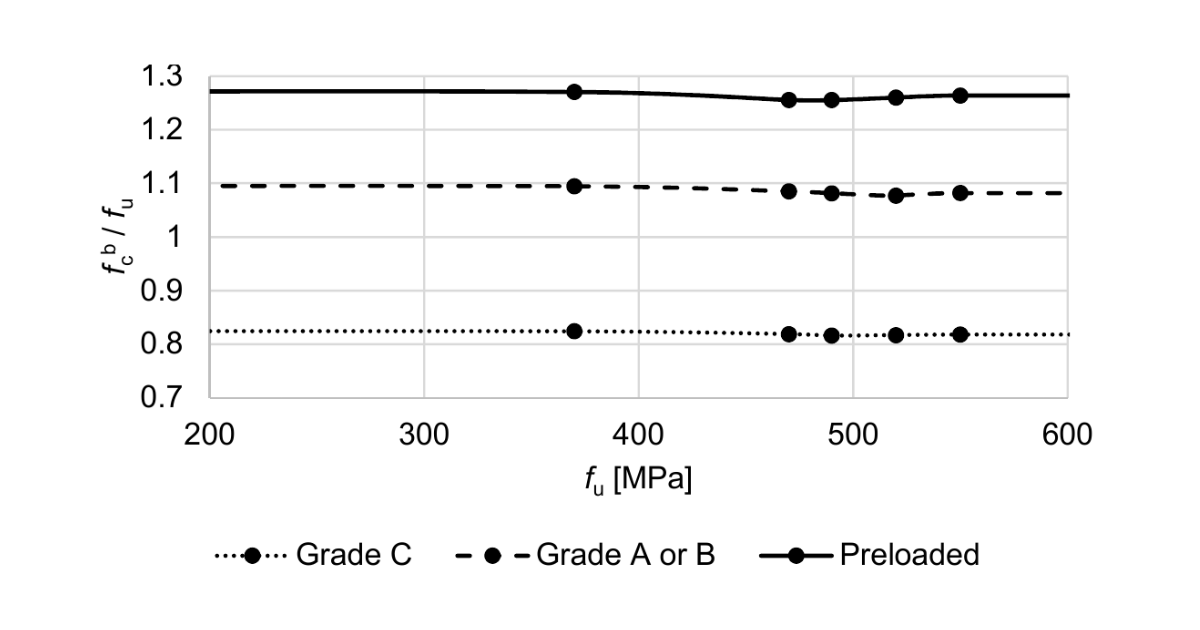### Choose language# Code-check of preloaded bolts according to Indian standards

Preloaded bolts are checked for shear slip resistance and combined tension and shear.
$$Slip resistanceSlip resistance of preloaded bolt is checked according to IS 800, Cl. 10.4.3:$V_{sf} \le V_{dsf}$where: $$V_{dsf} = V_{nsf} / \gamma_{mf}$$ – design shear capacity of a bolt as governed by slip for friction type connection $$V_{nsf} = \mu_f n_e K_h F_0$$ – nominal shear capacity of a bolt as governed by slip for friction type connection $$\mu_f$$ – coefficient of friction (slip factor) as specified in IS 800, Table 20; editable in Code setup $$n_e = 1$$ – number of effective interfaces offering frictional resistance to slip; each shear plane is checked separately $$K_h$$ – factor for bolt holes; $$K_h = 1.0$$ for fasteners in standard holes, $$K_h = 0.85$$ for fasteners in oversized and short slotted holes, $$K_h = 0.7$$ for fasteners in long slotted holes $$\gamma_{mf}$$ – partial safety factor for bolts – friction type – IS 800, Table 5, $$\gamma_{mf}=1.10$$ if slip resistance is designed at service load, $$\gamma_{mf}= 1.25$$ if slip resistance is designed at ultimate load; editable in Code setup $$F_0 = A_n f_0$$ – minimum bolt tension (proof load) at installation $$A_n$$ – net tensile stress area of the bolt $$f_0 = 0.7 f_{ub}$$ – proof stress Capacity after slipping (IS 800, Cl. 10.4.4) should be checked by switching bolt type from friction to bearing – tension/shear interaction for design capacity at ultimate load.Tension capacity of boltsA bolt subjected to a factored tensile force is checked according to IS 800, Cl. 10.3.5:$T_f \le T_{df}$where: $$T_{df} = T_{nf} / \gamma_{mf}$$ – design tensile capacity of the friction bolt $$T_{nf} = \min \{ 0.9 f_{ub} A_n, \, f_{yb} A_s (\gamma_{mf} / \gamma_{m0}) \}$$ – nominal tensile capacity of the friction bolt $$f_{ub}$$ – ultimate tensile strength of the bolt $$f_{yb}$$ – yield strength of the bolt $$A_n$$ – net tensile stress area of the bolt $$A_s$$ – cross-section area at the shank $$\gamma_{mf}$$ – partial safety factor for bolts – friction type – IS 800, Table 5, $$\gamma_{mf}=1.10$$ if slip resistance is designed at service load, $$\gamma_{mf}= 1.25$$ if slip resistance is designed at ultimate load; editable in Code setup $$\gamma_{m0} = 1.1$$ – partial safety factor for resistance governed by yielding – IS 800, Table 5; editable in Code setup Prying forces are determined by finite element analysis and are included in the tensile force.Friction bolt subjected to combined shear and tensionA bolt required to resist both design shear force and design tensile force at the same time shall according to IS 800, Cl. 10.3.6 satisfy:$\left( \frac{V_{sf}}{V_{df}} \right)^2 + \left( \frac{T_{f}}{T_{df}} \right)^2 \le 1.0$where: $$V_{sf}$$ – applied factored shear at design load $$V_{df}$$ – design shear strength $$T_f$$ – externally applied factored tension at design load $$T_{df}$$ – design tension strength$$